## Whatis ODE?

Ordinary differential equation (ODE) is an equation that involves a rate of change of a dependent variable with respect to one independent variable. The rate of change is denoted by one of these equal notations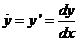. Suppose we have a function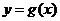, then variable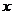is called domain or independent variable and variable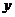is called range or dependent variable.

Because the rate change is involved only one variable, we call it ordinary derivative. This is in contrast to partial derivatives that involves rate of change of several variables. That is why we have ordinary differential equation (ODE) in contrast to partial differential equation (PDE).

Example: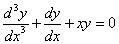is an ordinary differential equation with respect to one variable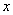. The ODE is said to have 3-rd order because the highest order of the rate is 3. It is a first degree ODE because the power of the highest order is 1.

The following example illustrates the meaning of solution to differential equation.

Example:
Suppose we have a function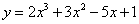. Differentiate this equation gives an ODE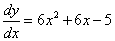. Thus the general solution of ODEis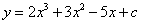. When we know the value of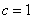, we say that the particular solution of the ODE is. Notice that we reverse the strategy from solution to equation.

The general equation of the first order Ordinary Differential Equation (ODE) is given as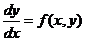The right hand side is a function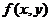, which usually may involve the independent variable, the dependent variableand constants. Sometimes, either variableorare constant and it may simplify the case. In case the dependent variableis not presence, the right hand side becomes function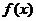and the solution of differential equation can be solved immediately by integration.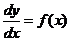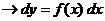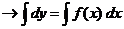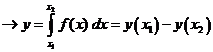Example below illustrates a case when both variableorare constants such that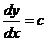.

Example:
Suppose we found that population of our region has a constant rate of growth. If we put notation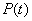for the number of population in the region at time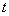(measured in year), we can equate a constant growth rate with equation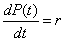. Suppose we know that the growth rate is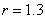and this year population is 1200 people. What is the number of population 10 years from now? To answer that question we need to solve the ODE, which will give answer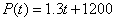, inputting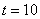gives answer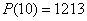When the dependent variablein the right hand side of general ODE equationis not a constant, the integration is not straightforward as above example. It needs differential algebraic equation as shown in the example below.

Example:

Solve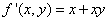for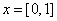with initial value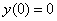As you may have learn in Calculus, we can write the equation as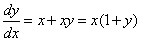. We can separateand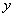such that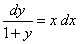. Integrate both side of the equation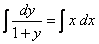we get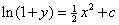or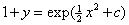, or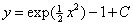. We can input initial conditionintowe get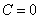. Thus, the solution is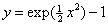. We plot for the range of x between 0 and 1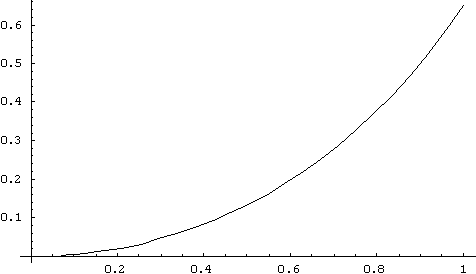Preferable reference for this tutorial is

Teknomo, Kardi (2015) Solving Ordinary Differential Equation (ODE). https:\\people.revoledu.com\kardi\tutorial\ODE\